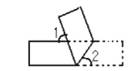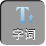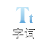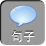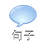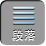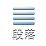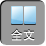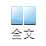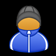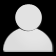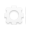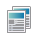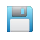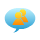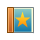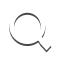-AA+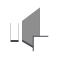1、如图 1，将三角形纸片沿 DE折叠，使点 A落在 BC上。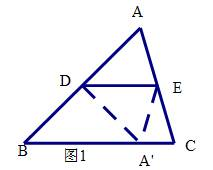1）若 AB= 4A D= 2，则 BD=________;

2）若∠ ADA= 800，∠ AEA= 700，则∠ ADE=________,∠ AED=__________,∠ A=___________.

∴⊿ ADE≌⊿ A DE,

AD= A D= 2

BD= AB-AD= 4-2= 2

( 2)∵⊿ ADE≌⊿ A DE

∴∠ AED=∠ A ED

2AED= 2A ED=∠ AEA

∴∠ AED= 350

∴∠ ADE=∠ A DE= 400

A= 180 -AED -ADE= 1050

( 1)如图 2DAB边上的中点，将⊿ ABC沿过 D的直线折叠，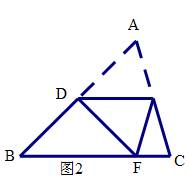2、如图，将一张矩形纸片 ABCD沿 EF折叠，使顶点 CD分别落在点 C′， D′处， CEAF于点 G，若∠ CEF= 70°，则∠ GFD′=？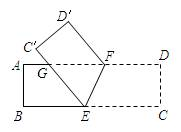∵∠ CEF= 70°，

∴∠ EFG=∠ CEF= 70°，

∴∠ EFD= 180° -70°= 110°，

∴∠ GFD′=∠ EFD-EFG

= 110° -70°，

= 40°．

3、将一张矩形纸条 ABCD按如图所示折叠，若∠ FEC= 64°．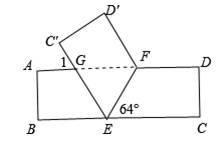①求∠ 1的度数；②求证：△ EFG是等腰三角形

∴∠ BEG= 180° -64°× 2= 52°

ADBC

∴∠ 1=∠ BEG= 52°

②证明：∵ ADBC

∴∠ GFE=∠ FEC

∴∠ GEF=∠ GFE

GE= GF

∴△ EFG是等腰三角形

1）将一长方形纸条按如图所示折叠，∠ 2= 54°，则∠ 1=？